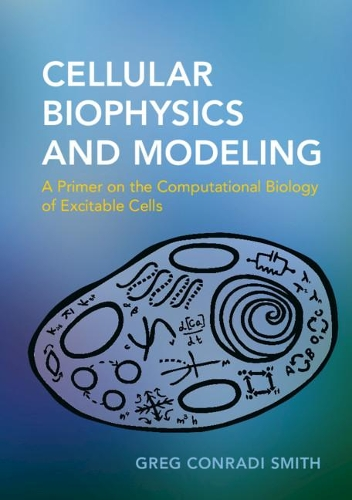•Cellular Biophysics and Modeling: A Primer on the Computational Biology of Excitable Cells (Paperback)

(author)
£38.99
Paperback 394 Pages / Published: 14/03/2019
• In stock online
• Free UK delivery

Usually dispatched within 24 hours

What every neuroscientist should know about the mathematical modeling of excitable cells. Combining empirical physiology and nonlinear dynamics, this text provides an introduction to the simulation and modeling of dynamic phenomena in cell biology and neuroscience. It introduces mathematical modeling techniques alongside cellular electrophysiology. Topics include membrane transport and diffusion, the biophysics of excitable membranes, the gating of voltage and ligand-gated ion channels, intracellular calcium signalling, and electrical bursting in neurons and other excitable cell types. It introduces mathematical modeling techniques such as ordinary differential equations, phase plane, and bifurcation analysis of single-compartment neuron models. With analytical and computational problem sets, this book is suitable for life sciences majors, in biology to neuroscience, with one year of calculus, as well as graduate students looking for a primer on membrane excitability and calcium signalling.

Publisher: Cambridge University Press
ISBN: 9780521183055
Number of pages: 394
Weight: 780 g
Dimensions: 246 x 174 x 20 mm

MEDIA REVIEWS
'In this text, Conradi Smith does an excellent job of teaching students with no mathematical training beyond calculus how to use differential equations to understand the basic principles of cell physiology and excitability. He skilfully walks students through the steps of modeling and analysis, all the while working to develop intuition and insight into how things work. His emphasis on computational methods for solution as well as graphical and geometrical means for interpretation enables him to communicate complex ideas in understandable ways. Furthermore, his patience and attention to detail will be appreciated by those students who have not had extensive exposure to the art of mathematical modeling. This text is a wonderful addition to the mathematical biology textbook literature.' James P. Keener, University of Utah
'In this text, Conradi Smith does an excellent job of teaching students with no mathematical training beyond calculus how to use differential equations to understand the basic principles of cell physiology and excitability. He skilfully walks students through the steps of modeling and analysis, all the while working to develop intuition and insight into how things work. His emphasis on computational methods for solution as well as graphical and geometrical means for interpretation enables him to communicate complex ideas in understandable ways. Furthermore, his patience and attention to detail will be appreciated by those students who have not had extensive exposure to the art of mathematical modeling. This text is a wonderful addition to the mathematical biology textbook literature.' James P. Keener, University of Utah